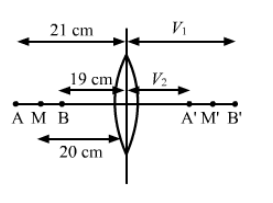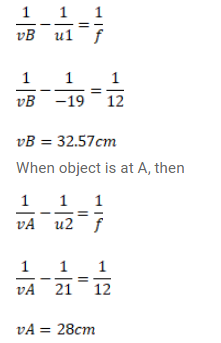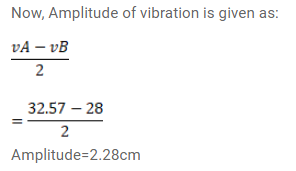# A particle executes a simple harmonic motion of`
Question:

A particle executes a simple harmonic motion of amplitude $1.0 \mathrm{~cm}$ along the principal axis of a convex lens of focal length $12 \mathrm{~cm}$. The mean position of oscillation is at $20 \mathrm{~cm}$. from the lens. Find the amplitude of oscillation of the image of the particle.

Solution: